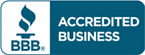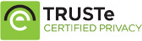# Solution manual for Numerical Methods for Engineers 7th edition by Steven C Chapra

\$30.00

Numerical Methods for Engineers 7th edition by Steven C Chapra Answers to questions

Full Chapters are included## Solution manual for Numerical Methods for Engineers 7th edition by Steven C Chapra

Table of Contents Solution manual for Numerical Methods for Engineers 7th edition by Steven C Chapra

Part 1 Modeling, Computers, and Error Analysis
Chapter 1 Mathematical Modeling and Engineering Problem Solving
Chapter 2 Programming and Software
Chapter 3 Approximations and Round-Off Errors
Chapter 4 Truncation Errors and the Taylor Series
Part 2 Roots of Equations
Chapter 5 Bracketing Methods
Chapter 6 Open Methods
Chapter 7 Roots of Polynomials
Chapter 8 Case Studies: Roots of Equations
Part 3 Linear Algebraic Equations
Chapter 9 Gauss Elimination
Chapter 10 LU Decomposition and Matrix Inversion
Chapter 11 Special Matrices and Gauss-Seidel
Chapter 12 Case Studies: Linear Algebraic Equations
Part 4 Optimization
Chapter 13 One-Dimensional Unconstrained Optimization
Chapter 14 Multidimensional Unconstrained Optimization
Chapter 15 Constrained Optimization
Chapter 16 Case Studies: Optimization
Part 5 Curve Fitting
Chapter 17 Least-Squares Regression
Chapter 18 Interpolation
Chapter 19 Fourier Approximation
Chapter 20 Case Studies: Curve Fitting
Part 6 Numerical Differentiation and Integration
Chapter 21 Newton-Cotes Integration Formulas
Chapter 22 Integration of Equations
Chapter 23 Numerical Differentiation
Chapter 24 Case Studies: Numerical Integration and Differentiation
Part 7 Ordinary Differential Equations
Chapter 25 Runge-Kutta Methods
Chapter 26 Stiffness and Multistep Methods
Chapter 27 Boundary-Value and Eigenvalue Problems
Chapter 28 Case Studies: Ordinary Differential Equations
Part 8 Partial Differential Equations
Chapter 29 Finite Difference: Elliptic Equations
Chapter 30 Finite Difference: Parabolic Equations
Chapter 31 Finite-Element Method
Chapter 32 Case Studies: Partial Differential Equations
Appendix A The Fourier Series
Appendix B Getting Started with Matlab
Appendix C Getting Started with Mathcad
Bibliography
Index of Solution manual for Numerical Methods for Engineers 7th edition by Steven C Chapra

## Reviews

There are no reviews yet.